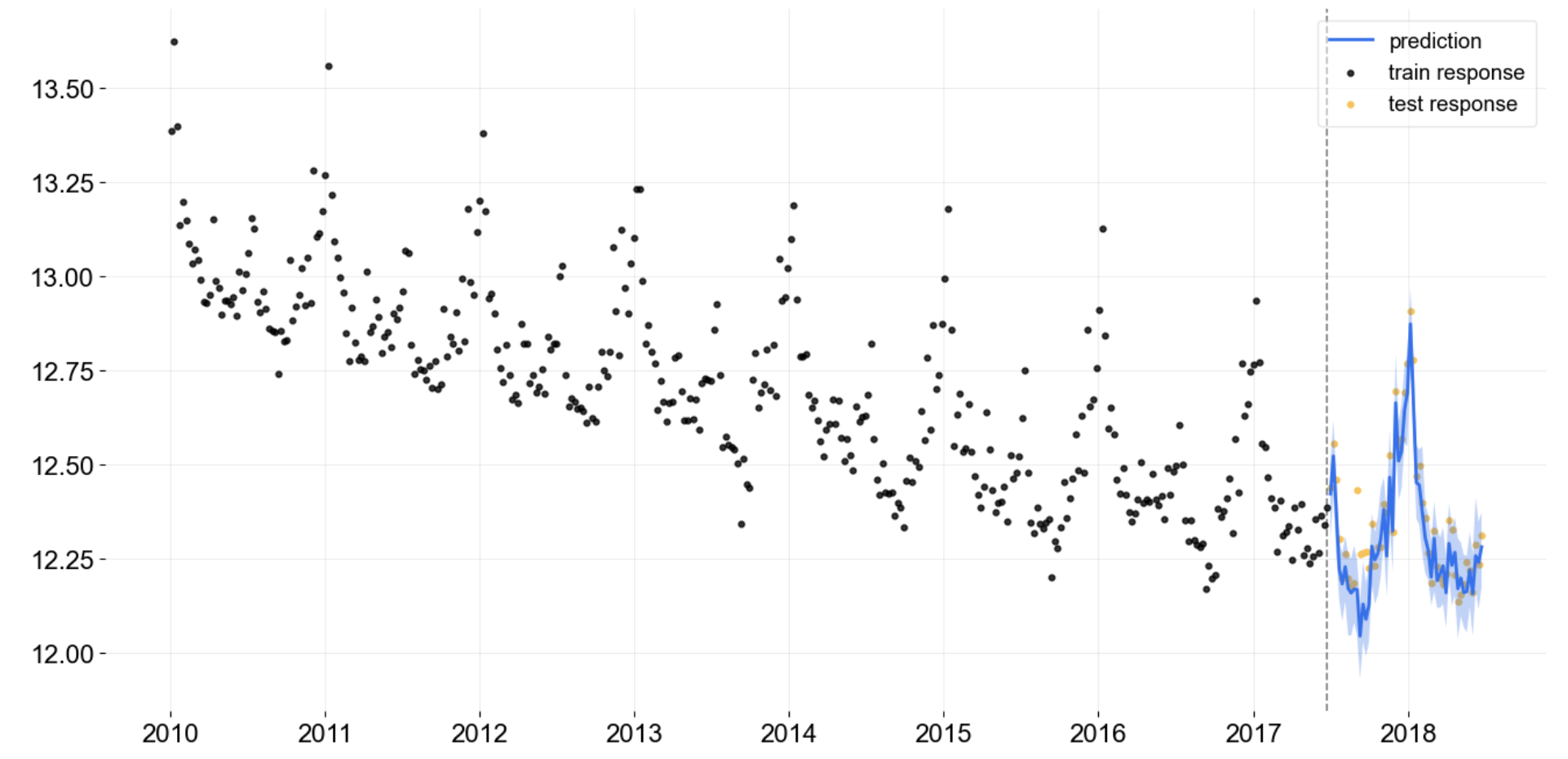# Disclaimer

This project

• is stable and being incubated for long-term support. It may contain new experimental code, for which APIs are subject to change.

# Orbit: A Python Package for Bayesian Forecasting

Orbit is a Python package for Bayesian time series forecasting and inference. It provides a familiar and intuitive initialize-fit-predict interface for time series tasks, while utilizing probabilistic programming languages under the hood.

For details, check out our documentation and tutorials:

Currently, it supports concrete implementations for the following models:

• Exponential Smoothing (ETS)
• Damped Local Trend (DLT)
• Local Global Trend (LGT)
• Kernel Time-based Regression (KTR-Lite)

It also supports the following sampling methods for model estimation:

• Markov-Chain Monte Carlo (MCMC) as a full sampling method
• Maximum a Posteriori (MAP) as a point estimate method
• Variational Inference (VI) as a hybrid-sampling method on approximate distribution

## Installation

### Installing Stable Release

Install from PyPi:

``````\$ pip install orbit-ml
``````

Install from source:

``````\$ git clone https://github.com/uber/orbit.git
\$ cd orbit
\$ pip install -r requirements.txt
\$ pip install .
``````

### Installing from Dev Branch

``````\$ pip install git+https://github.com/uber/[email protected]
``````

### FULL Bayesian Prediction

``````from orbit.utils.dataset import load_iclaims
from orbit.models.dlt import DLTFull
from orbit.diagnostics.plot import plot_predicted_data

# log-transformed data
# train-test split
test_size = 52
train_df = df[:-test_size]
test_df = df[-test_size:]

dlt = DLTFull(
response_col='claims', date_col='week',
regressor_col=['trend.unemploy', 'trend.filling', 'trend.job'],
seasonality=52,
)
dlt.fit(df=train_df)

# outcomes data frame
predicted_df = dlt.predict(df=test_df)

plot_predicted_data(
training_actual_df=train_df, predicted_df=predicted_df,
date_col=dlt.date_col, actual_col=dlt.response_col,
test_actual_df=test_df
)
``````## Demo

Forecasting / Nowcasting with Regression in DLT:Backtest on M3 Data:More examples can be found under tutorials and examples.

# Contributing

We welcome community contributors to the project. Before you start, please read our code of conduct and check out contributing guidelines first.

# Versioning

We document versions and changes in our changelog.

# References

## Presentations

Check out the deck we presented in a meet up event in Jul. 2021.

## Citation

To cite Orbit in publications, refer to the following whitepaper:

Orbit: Probabilistic Forecast with Exponential Smoothing

Bibtex:

``````@misc{
ng2020orbit,
title={Orbit: Probabilistic Forecast with Exponential Smoothing},
author={Edwin Ng,
Zhishi Wang,
Huigang Chen,
Steve Yang,
Slawek Smyl},
year={2020}, eprint={2004.08492}, archivePrefix={arXiv}, primaryClass={stat.CO}
}
``````

## Papers

• Bingham, E., Chen, J. P., Jankowiak, M., Obermeyer, F., Pradhan, N., Karaletsos, T., Singh, R., Szerlip, P., Horsfall, P., and Goodman, N. D. Pyro: Deep universal probabilistic programming. The Journal of Machine Learning Research, 20(1):973–978, 2019.
• Hoffman, M.D. and Gelman, A. The No-U-Turn sampler: adaptively setting path lengths in Hamiltonian Monte Carlo. J. Mach. Learn. Res., 15(1), pp.1593-1623, 2014.
• Hyndman, R., Koehler, A. B., Ord, J. K., and Snyder, R. D. Forecasting with exponential smoothing: the state space approach. Springer Science & Business Media, 2008.
• Smyl, S. Zhang, Q. Fitting and Extending Exponential Smoothing Models with Stan. International Symposium on Forecasting, 2015.

## Related projects

Get A Weekly Email With Trending Projects For These Topics
No Spam. Unsubscribe easily at any time.
Python (1,137,692
Time Series (1,926
Regression (1,307
Forecasting (469
Bayesian (371
Stan (279
Forecast (260
Probabilistic Programming (204
Arima (126
Related Projects